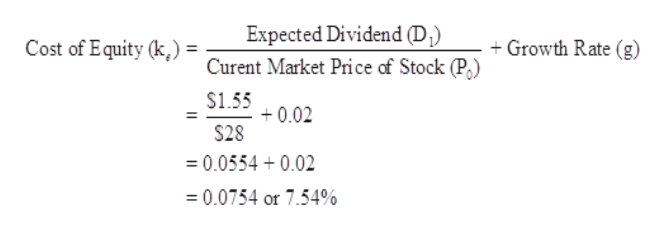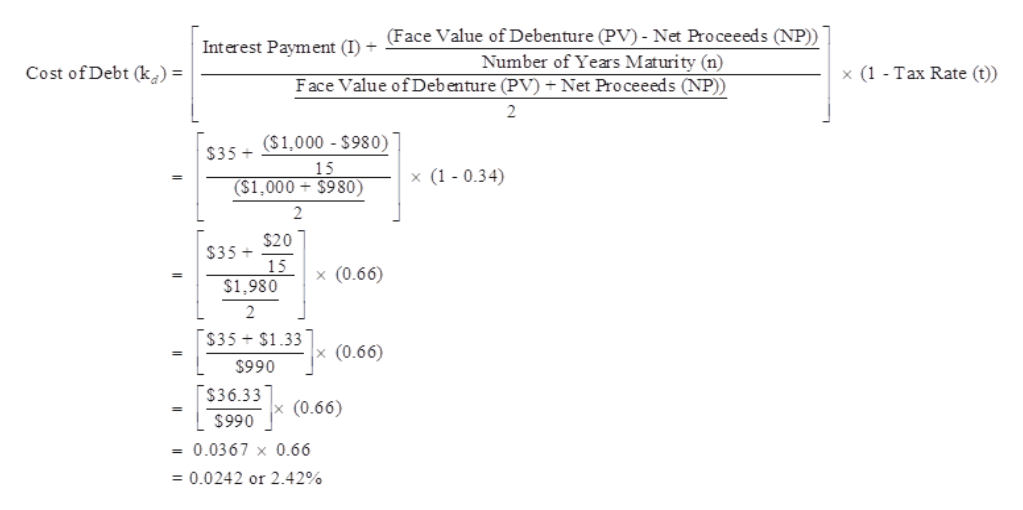# R.S. Green has 250,000 shares of common stock outstanding at a market price of \$28 a share. Next year's annual dividend is expected to be \$1.55 a share. The dividend growth rate is 2 percent. The firm also has 7,500 bonds outstanding with a face value of \$1,000 per bond. The bonds carry a 7 percent coupon, pay interest semiannually, and mature in 7.5 years. The bonds are selling at 98 percent of face value. The company's tax rate is 34percent. What is the firm's weighted average cost of capital

Question
270 views

R.S. Green has 250,000 shares of common stock outstanding at a market price of \$28 a share. Next year's annual dividend is expected to be \$1.55 a share. The dividend growth rate is 2 percent. The firm also has 7,500 bonds outstanding with a face value of \$1,000 per bond. The bonds carry a 7 percent coupon, pay interest semiannually, and mature in 7.5 years. The bonds are selling at 98 percent of face value. The company's tax rate is 34
percent. What is the firm's weighted average cost of capital

check_circle

Step 1

Cost of Equity:

Cost of equity is the amount or rate which a company bears for the issue of share. In other words, it is amount which a company give it equity shareholders as dividend. It is denoted by ke.

Cost of Debt:

Cost of Debts is the amount or rate which a company bears on the borrowings. It also stated as interest on loan and debts. It is calculated on the earning or profit before tax because interest on borrowing is a deductible amount from income tax. It is denoted by kd.

Weighted Average Cost of Capital (WACC):

WACC is calculation in which a firm’s cost of capital weighted according to their proportion. It is denoted by ko.

Step 2

Given,

Current Market Price of Stock (P0) = \$28

Expected Dividend (D1) per Stock = \$1.55

Growth Rate (g) = 2% or 0.02

Calculation of Cost of Equity (Ke):help_outlineImage TranscriptioncloseExpected Dividend (D) Curent Market Price of Stock (P) Cost of Equity (k,) = Growth Rate (g) \$1.55 0.02 \$28 0.0554 0.02 0.0754 or 7.54% fullscreen
Step 3

Given,

Face Value of Debenture = \$1,000

Coupon Rate = 7% or 0.07

Interest Payment (I) = (Face Value of Debenture * Coupon Rate) / 2

= (\$1,000 * 0.07) / 2

= \$70 / 2

= \$35

Se...help_outlineImage Transcriptionclose(Face Value of Debenture (PV) - Net Proceeeds (NP)) Interest Payment (I) + Number of Years Maturity (n) Cost of Debt (k) = x (1 - Tax Rate (t) Face Value ofDebenture (PV) Net Proceeeds (NP)) 2 (\$1,000 \$980) \$35+ 15 x (1 - 0.34) (\$1,000 \$980) 2 \$20 \$35+ 15 \$1,980 x (0.66) 2 \$35 \$1.33 (0.66) \$990 \$36.33 (0.66) \$990 0.0367 x 0.66 = 0.0242 or 2.42% fullscreen

### Want to see the full answer?

See Solution

#### Want to see this answer and more?

Solutions are written by subject experts who are available 24/7. Questions are typically answered within 1 hour.*

See Solution
*Response times may vary by subject and question.
Tagged in